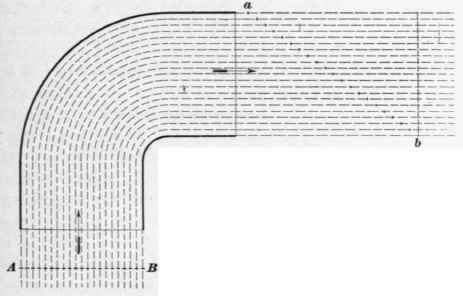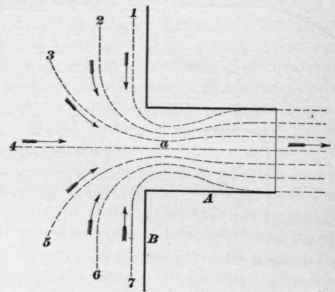43. The resistance which is encountered by air and other fluids in moving through pipes, flues, and conduits is usually spoken of as friction, although the term, as thus used, is not strictly accurate.

The resistance arises from several causes:

1. Skin friction, which is the actual friction of the fluid against the surfaces over which it passes.

2. The abrupt changes of direction of the current, at elbows and tees.

3. The movement of one part of the current upon another, in passing around curves.

4. The abrupt changes in velocity, which occur at pockets and enlargements.

5. The formation of whirls or eddies. G. The interference of currents.

## 44. Skin Friction

Skin Friction. The resistance due to skin friction varies (1) directly as the length of the pipe or flue; (2) inversely as the diameter or length of a side; (3) directly as the square of the velocity. Hence, other things being equal, the pipe should be as short as possible; its diameter should be as large as possible, and the quantity passing through it in a given time should be as small as circumstances will permit. The last consideration is the most important of all, since, if the quantity passing through a given pipe is doubled, the velocity is also doubled, and the resistance is increased 22, or 4 times.

The condition of the pipe itself, whether smooth or rough, also affects the force required to overcome the friction, a smooth pipe offering less resistance than a rough one. Hence, metal pipes are to be preferred, in this respect, to brick conduits. A polished pipe offers less resistance than a smooth one not polished. A pipe or flue having its inside covered with soot offers much greater resistance than a similar pipe that is clean.

## 45. Resistance From Changes Of Direction

Resistance From Changes Of Direction. When a current of air encounters an obstacle, the particles composing it are compelled to deflect from their normal direction and to move sideways sufficiently to pass by the obstruction, consequently the current loses some of its kinetic energy.fig. a.Fig. 4.

When a current is compelled to change its direction abruptly, the particles tend to move as shown in Fig. 3. An area of high pressure will be formed at a, and another of correspondingly low pressure at b. The effective area of the pipe or conduit will be reduced at c, and the velocity of the fluid will be greater at that point than at e and d. Thus, the force which is spent in maintaining the abnormal pressures at a and b, and in imparting the increase of velocity at c, is all wasted.Fig. 5.

## 46. Effects Produced By-Curves

Effects Produced By-Curves. If the turn is less abrupt, as in Fig. 4, the waste of energy is considerably reduced. The tendency to form high and low pressure areas, as in Fig. 3, is nearly destroyed. But the particles of the fluid are still compelled to slide over one another. Thus, the particles at A and B will reach the points a and b, respectively, at the same time, if they travel equal distances in equal time. The power which is expended in sliding the particles of fluid upon one another is wasted.Fig. 6.

This loss cannot be avoided, whether the turn be made sharply, as in Fig. 3, on a small radius, as in Fig. 4, or on a long radius, as in Fig. 5. But the time which is afforded for making the change of direction (the velocity being the same in each case) is much greater in Fig. 5 than in either of the others. The force which is required to deflect a moving body from its normal path is inversely proportional to the square of the time in which the change of direction is made. Hence, if the change of direction is made in 2 seconds in Fig. 4, and in 4 seconds in Fig. 5, then 42 ÷22 = 4 times as much force will be required when turning the curve of shorter radius.Fig. 7.

## 47. Interference Of Currents

Interference Of Currents. The effect of opposing currents is shown in Fig. 6. This illustrates the formation of what is called the contracted vein. The branch A is attached at right angles to a tank or reservoir B. It will be seen that the particles which move on the lines 1 and 7 would collide if their motion were continued, and would destroy each other's motion. Their energy is spent, however, in deflecting the other parts of the current somewhat out of their course. The effect of the opposition of the various currents is to reduce the effective diameter of the stream at a to less than the diameter of the branch A. Consequently, the amount of fluid which will be delivered through the branch will be less than it should be. The trouble may be remedied by providing the branch with a mouthpiece which is curved so as to properly guide the' parts of the current, as shown in Fig. 7. The branch will then discharge to its full capacity.Fig. 8.